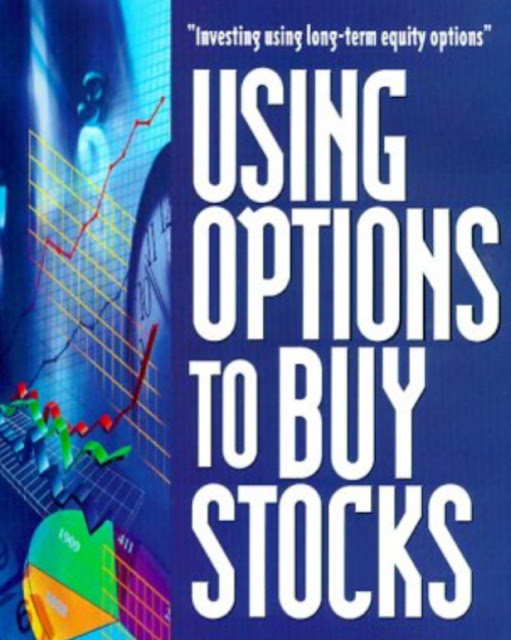## Posts

Showing posts with the label volatility-calculation

### VOLATILITY AND PREMIUMS- Measuring VolatilityVOLATILITY AND PREMIUMS Measuring Volatility Introduction The volatility of the underlying stock is extremely important in the world of options because, among other things, it determines the likelihood that an option will wind up in or out of the money by the expiration date. This chapter describes the procedure by which volatility is numerically calculated and shows how to calculate the chances that a given LEAP option will wind up in or out of the money by its expiration date. To begin, suppose that: P is the price of the underlying issue S is the strike (exercise price) of the option r is the risk-free interest rate in decimal form t is the time in years till expiration v is the annualized volatility in decimal form δ the annualized dividend rate in decimal form Further, let h be given by: Then the probability of winding up in the money by the expiration date for a put option  is given by: The probability of winding up in the mone